# Robot Framework - Working With Variables

In this chapter, we will discuss how to create and use variables in Robot Framework. Variables are used to hold a value, which can be used in test cases, user-defined keywords, etc.

We are going to discuss following variables available in Robot Framework

• Scalar Variable
• List Variable
• Dictionary Variable

We will understand the working of each of this variable with the help of test cases in Ride.

## Scalar Variable

Scalar variables will be replaced with the value they are assigned. The syntax for scalar variable is as follows −

${variablename}  We can use scalar variable to store strings, objects, lists, etc. We will first create a simple test case and make use of scalar variable in it. Open RIDE using ride.py in the command line and create a new project.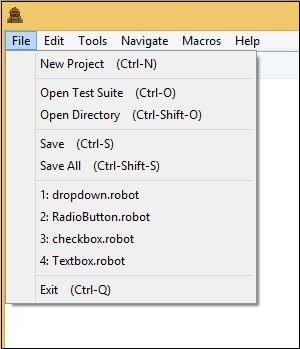Click New Project. Now, give a name to your project.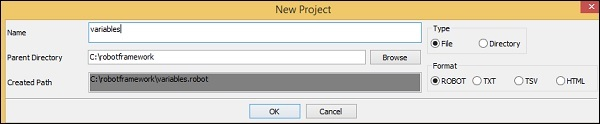The name given is variables. Click OK to save the project. Right-click on the name of the project created and click on New Test Case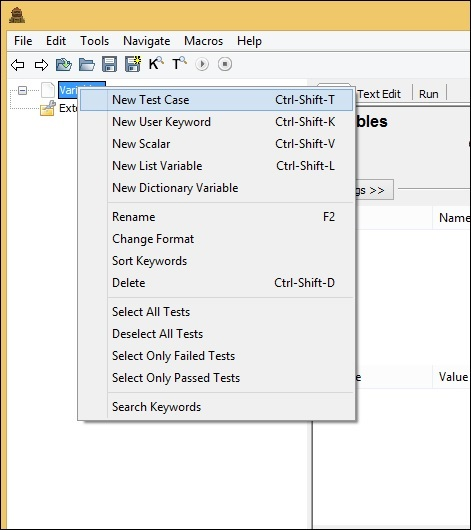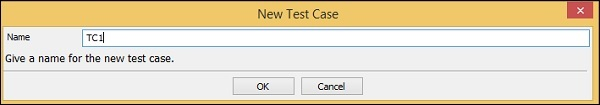Give a name to the test case and click OK. We are done with the project setup and now will write test cases for the scalar variables to be used in our test case. Since we need Selenium library, we need to import the same in our project. Click on your project on the left side and use Library from Add Import −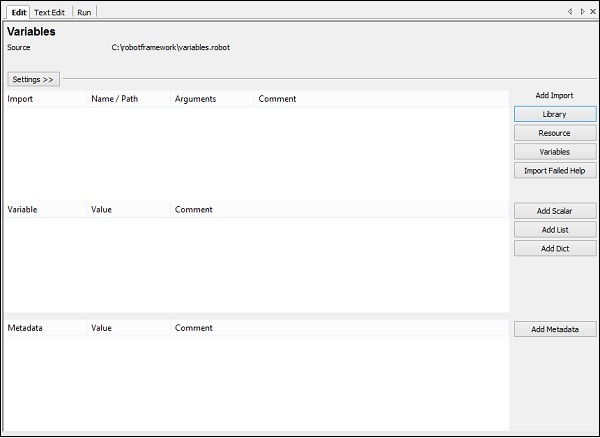Upon clicking Library, a screen will appear where you need to enter the library name −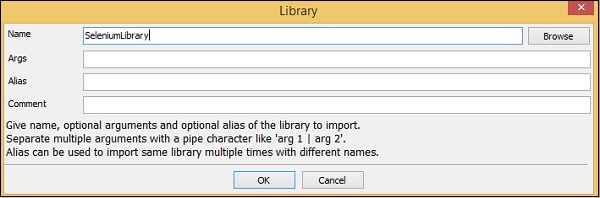Click OK and the library will get displayed in the settings.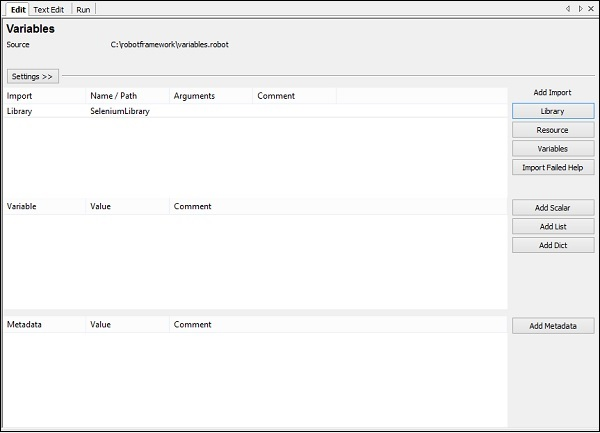The name given has to match with the name of the folder installed in site-packages. If the name does not match, the library name will be shown in red −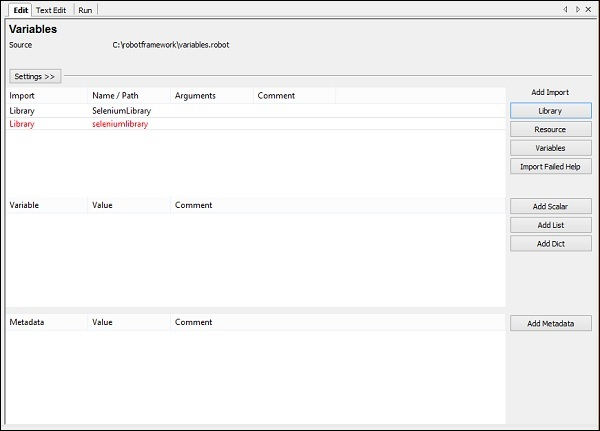## Test Case for Scalar Variable In the above test cases we hardcoded the values like the URL, email, password, which we are giving to the test case. The values used can be stored in a variable and instead of hardcoding, we can use the variable in those places.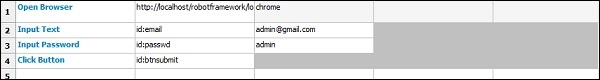To create scalar variable, right-click on your project and click on New Scalar as shown below −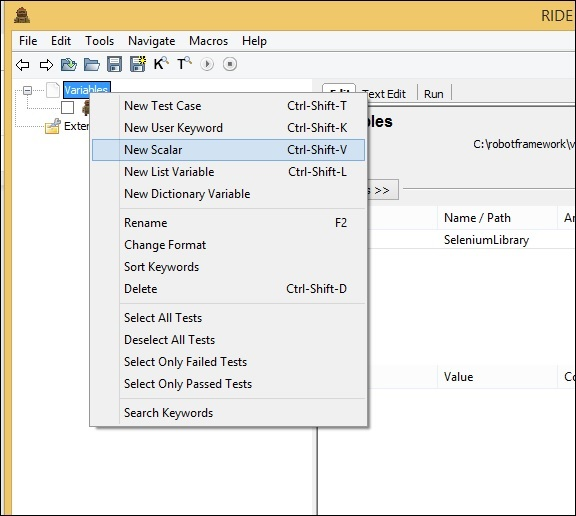Clicking on New Scalar will open the following screen to create the variable and the value we need to replace with when the variable in used inside test cases. We get${} for the Name field.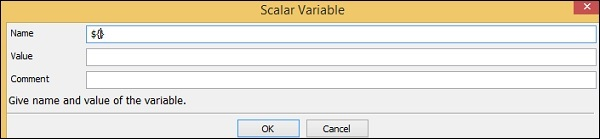Here we need to enter the name of the variable inside the curly braces as shown in the screen below −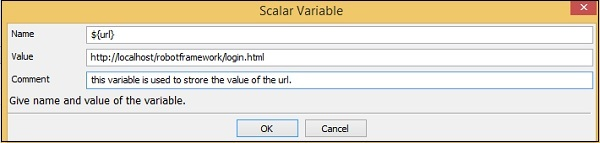The name of the variable is ${url}. The value is − http://localhost/robotframework/login.html. We added the comment as shown above. Click OK to save the scalar variable. The details of the variable are added as shown below −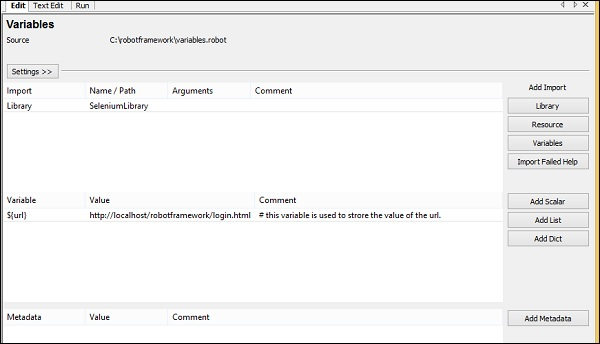The variable name is shown under the project created as follows −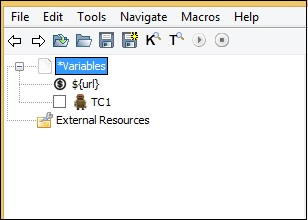Let us now use the scalar variable created inside our test case. ### Test case with URL hardcoded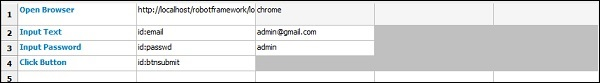In the above test case, we have to replace the URL with the variable we just created above. ### Test Case with Scalar Variable for URL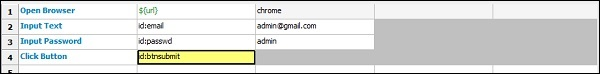Now, we will run the test case to see if it is taking the URL from the variable. Below is the output that we get when we run it. The URL http://localhost/robotframework/login.html is picked up from the scalar variable we created.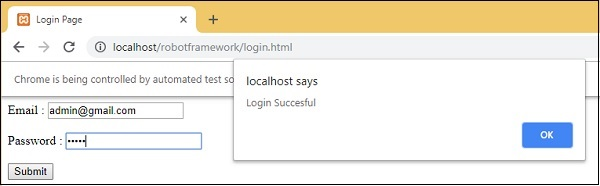### Execution Details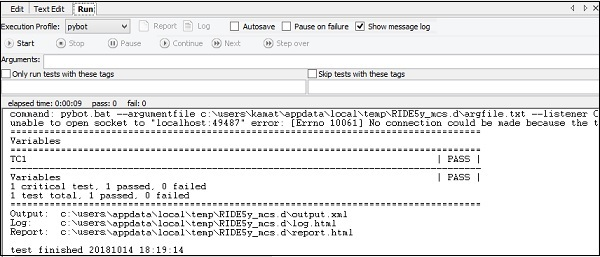The advantage of using variables is that you can change the value for that variable and it will be reflected in all test cases. You can use the variables in many test cases that you create under that project. Hardcoding of values can be a serious problem when you want to change something, you will have to go to individual test case and change the values for it. Having variables in one place gives us the flexibility to test the way we want with different values to the variables. Now, we will look into the next type of variable called the List variable. ## List Variable List variable will have an array of values. To get the value, the list item is passed as the argument to the list variable. ### Syntax @{variablename}  Suppose we have values A, B. To refer the values, we need to pass the list item as follows − @{variablename} // A @{variablename} // B  To add list variable, right-click on the project and click New List Variable.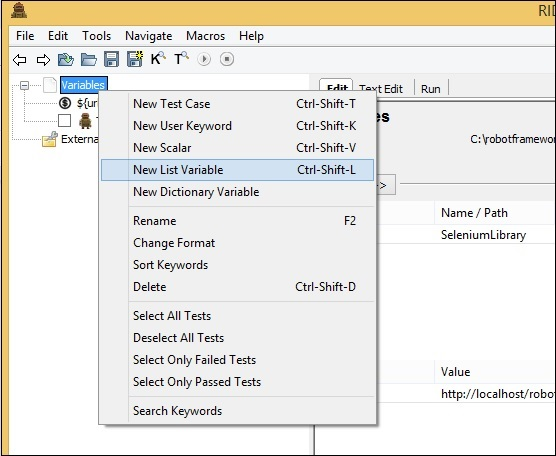Upon clicking New List Variable, a screen appears where we can enter the values −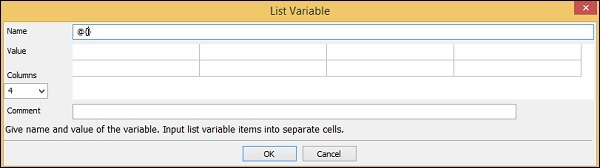The Name is given as @{} followed by Value. It also has 4 Columns selected. Right now, we will use just Column 1 and create the list variable, which will have values, email id and password as follows −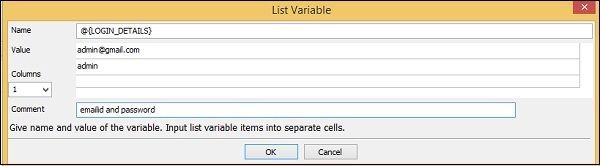The name of the list variable is @{LOGIN_DETAILS} and the values given are admin@gmail.com and admin, which has email id and password for the login page. Click OK to save the list variable. The variable is listed below the project as shown here −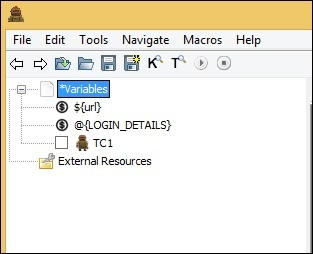The details of variables used are listed in the settings tab −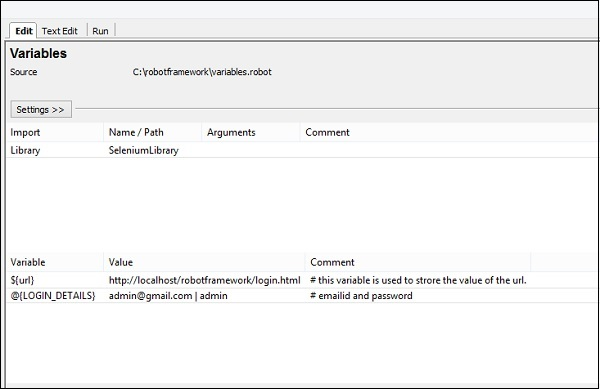Now, we will add the list variable inside the test cases as shown below. Here, we have hardcoded values for the Input Text and Password. Now, we will change it to use the list variable.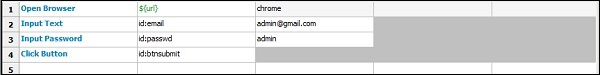Using List Variable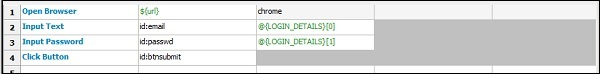Now, we will execute the test case to see if it is taking the values from the list variable −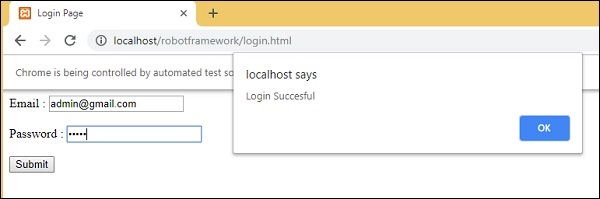It has taken the email id and password from the list variable as shown above in the test screen. The following screenshot shows the execution details for the same −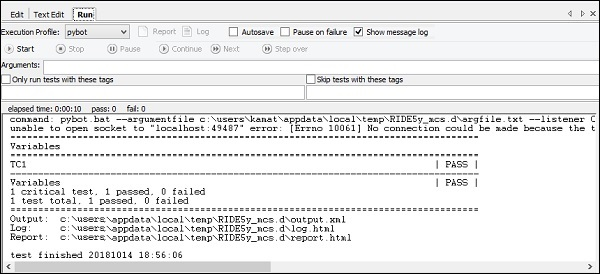In our next section, we will learn about the Dictionary Variable. ## Dictionary Variable Dictionary Variable is similar to list variable wherein we pass the index as an argument; however, in case of dictionary variable, we can store the details – key value form. It becomes easier to refer when used in the test case instead of using the index as 0, 1, etc. ### Syntax &{Variablename}  Suppose we are storing the values as key1=A, key2=B. It will be referred in the test case as − &{Variablename}[key1] // A &{Variablename}[key2] // B  Let us create dictionary variable in Ride. Right-click on Project and click on New Dictionary Variable.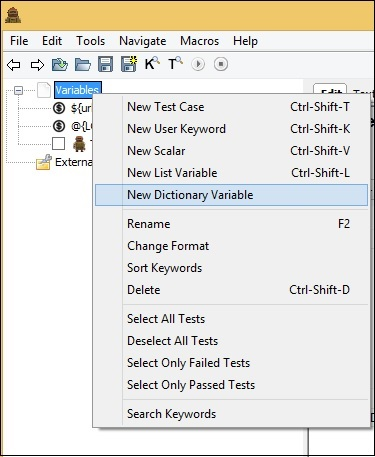Upon clicking New Dictionary Variable, a screen will appear as shown below −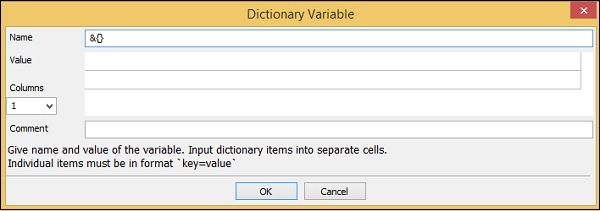The Name by default in the screen is &{} and it has Value and Columns option. We will enter the Name and the Values to be used in the test case.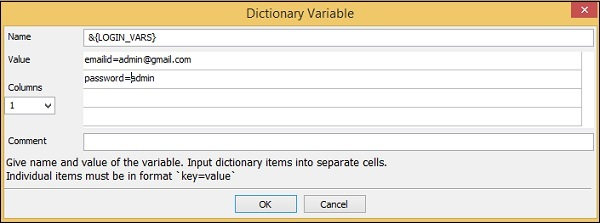Click OK to save the variable. The variable will be listed under the project and also in the settings as follows −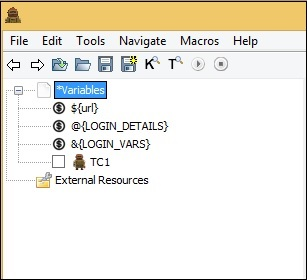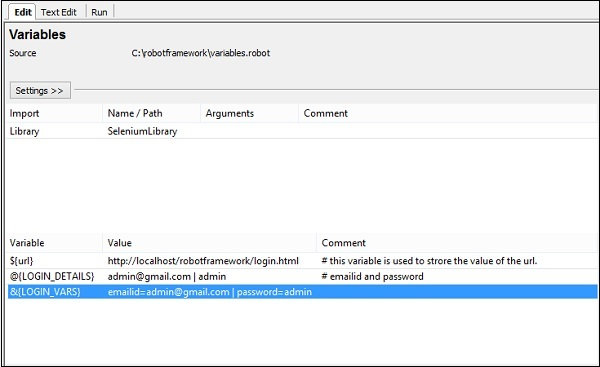We will change the test case to take the dictionary values.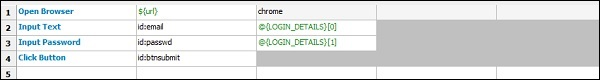We will change to dictionary variable as shown below. ### Using Dictionary Variable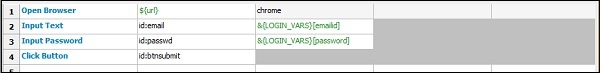Upon clicking run, we get the following −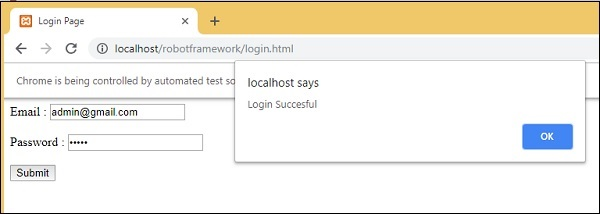The execution details are as follows −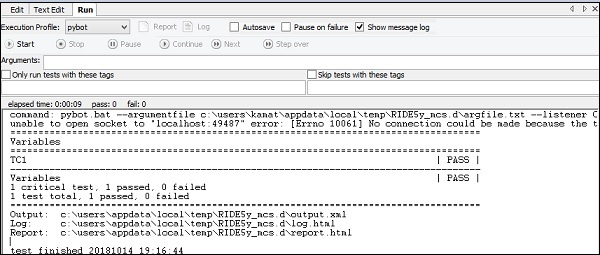We have seen the Edit and Run Tab so far. In case of TextEdit, we have the details of the test case written. We can also add variables required in TextEdit. ### Test case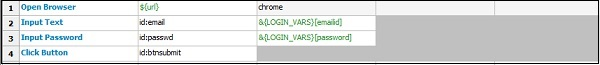We have used scalar variable and dictionary variable in the above test case. Here is the code so far in TextEdit; this is based on the test case written −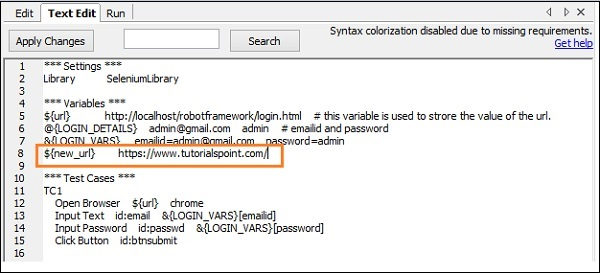The variables used are highlighted in Red. We can also create variables we want directly in TextEdit as shown below − We have added a scalar variable called${new_url} and the value given is https://www.tutorialspoint.com/.

Click Apply Changes button on the top left corner and the variable will be seen under the project as shown below −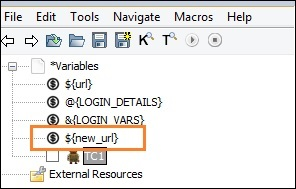Similarly, other variables − list and dictionary variables can be created directly inside TextEdit tab whenever required.

## Conclusion

We have seen how to create and use variables. There are three types of variables supported in robot framework − scalar, list and dictionary. We discussed in detail the working of all these variables.